# Newton's LawsPage 2

#### WATCH ALL SLIDES

Inertia

Slide 13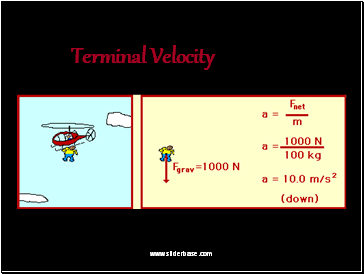Terminal Velocity

Slide 14## Newton’s Second Law

Force equals mass times acceleration.

F = ma

Slide 15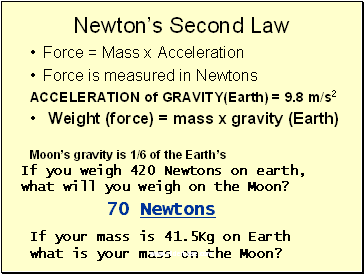Newton’s Second Law

Force = Mass x Acceleration

Force is measured in Newtons

ACCELERATION of GRAVITY(Earth) = 9.8 m/s2

Weight (force) = mass x gravity (Earth)

Moon’s gravity is 1/6 of the Earth’s

If you weigh 420 Newtons on earth, what will you weigh on the Moon?

70 Newtons

If your mass is 41.5Kg on Earth what is your mass on the Moon?

Slide 16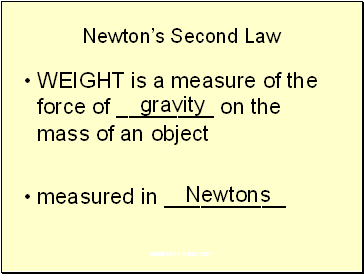Newton’s Second Law

WEIGHT is a measure of the force of on the mass of an object

measured in

gravity

Newtons

Slide 17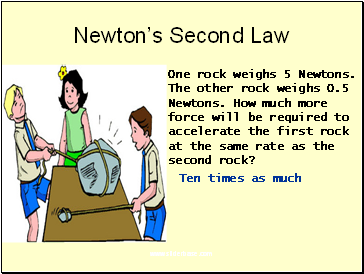Newton’s Second Law

One rock weighs 5 Newtons.

The other rock weighs 0.5

Newtons. How much more

force will be required to

accelerate the first rock

at the same rate as the second rock?

Ten times as much

Slide 18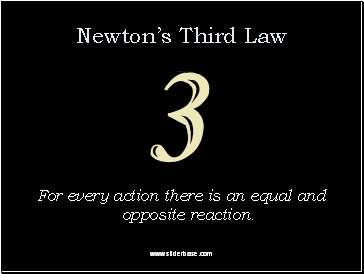## Newton’s Third Law

For every action there is an equal and opposite reaction.

Slide 19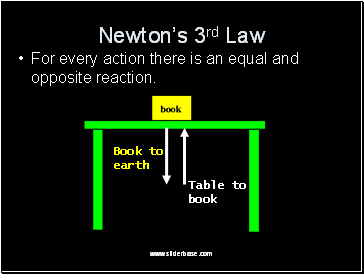Newton’s 3rd Law

For every action there is an equal and opposite reaction.

Book to

earth

Table to

book

Slide 20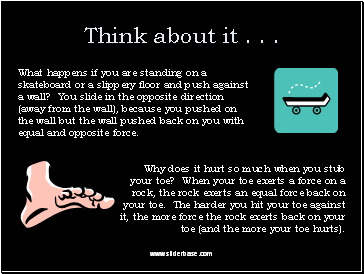Think about it . . .

What happens if you are standing on a skateboard or a slippery floor and push against a wall? You slide in the opposite direction (away from the wall), because you pushed on the wall but the wall pushed back on you with equal and opposite force.

Why does it hurt so much when you stub your toe? When your toe exerts a force on a rock, the rock exerts an equal force back on your toe. The harder you hit your toe against it, the more force the rock exerts back on your toe (and the more your toe hurts).

Slide 21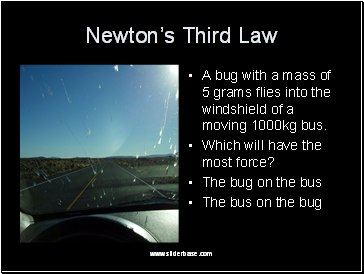Newton’s Third Law

A bug with a mass of 5 grams flies into the windshield of a moving 1000kg bus.

Which will have the most force?

The bug on the bus

The bus on the bug

Slide 22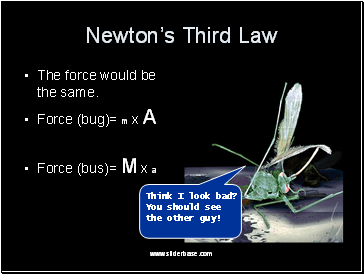Newton’s Third Law

The force would be the same.

Force (bug)= m x A

Force (bus)= M x a

You should see

the other guy!

Slide 23

Go to page:
1  2  3